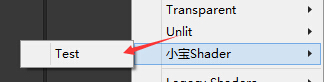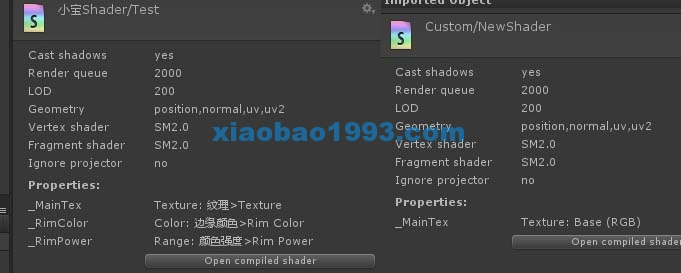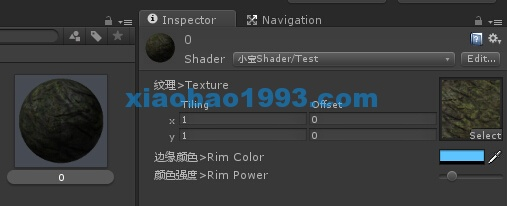```Shader "小宝Shader/Test" {
Properties {
_MainTex ("Base (RGB)", 2D) = "white" {}
}
//这里定义子着色器(一个子着色器渲染draw call)
//渲染类型为Opaque，不透明
Tags { "RenderType"="Opaque" }
LOD 200

//开始着色器编程语言段
CGPROGRAM

//使用兰伯特光照模式
#pragma surface surf Lambert

//变量声明
sampler2D _MainTex;

//输入结构
struct Input {
float2 uv_MainTex;
};

//函数的编写
void surf (Input IN, inout SurfaceOutput o) {
half4 c = tex2D (_MainTex, IN.uv_MainTex);
o.Albedo = c.rgb;
o.Alpha = c.a;
}
//结束着色器编程语言段
ENDCG
}
//“备胎”为普通漫反射 FallBack 音译为 “回滚”，俗称“备胎” 用来处理 设备老旧等导致Shader不能运行的情况
FallBack "Diffuse"
}
```## 基本框架

```Shader "小宝Shader/Test"
{
//属性
Properties
{
//纹理
_MainTex("基本纹理",2D)="White"{TexGen ObjectLinear}
}

//子着色器1
{
//通道
Pass
{
//设置纹理为属性中选择的纹理
SetTexture[_MainTex]{combine texture}
}

}

//备胎设为Unity自带的普通漫反射
Fallback" Diffuse "
}```

## 属性Properties

```//定义Shader属性
Properties {
_MainTex("纹理>Texture", 2D) = "white" {}//贴图
_RimColor("边缘颜色>Rim Color", Color) = (0.17, 0.36, 0.81, 0.0)//颜色
_RimPower("颜色强度>Rim Power", Range(0.6, 9.0)) = 1.0//定义浮点数属性
}```## 最后。Properties属性定义命名规则

Properties { Property [Property ...] }定义属性块，其中可包含多个属性，其定义如下

name ("display name", Range (minmax)) = number

name ("display name", Color) = (number,number,number,number)

name ("display name", 2D) = "name" { options }

name ("display name", Rect) = "name" { options }

name ("display name", Cube) = "name" { options }

name ("display name", Float) = number

name ("display name", Vector) = (number,number,number,number)

name 为变量名称 每一个变量名称都必须在前面加_下划线（不要问我为什么，规定就是这样。）;

(“display name” , variable)  “display name”为面板显示的名称 variable为变量的类型(类型如：Range(min,max),Color,2D,Rect,Cube,Float,Vector)

TexGen纹理生成类型。纹理的自动生成纹理坐标时的模式，可以是ObjectLinear, EyeLinear,SphereMap, CubeReflect, CubeNormal的其中之一;这些模式和OpenGL纹理生成模式相对应。注意如果使用自定义顶点程序，那么纹理生成将被忽略。